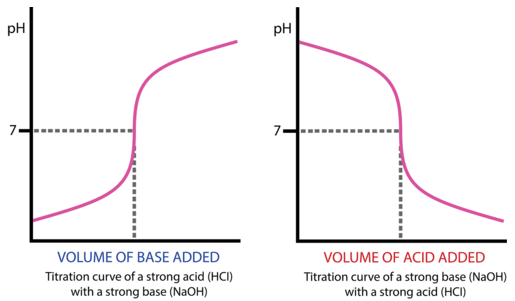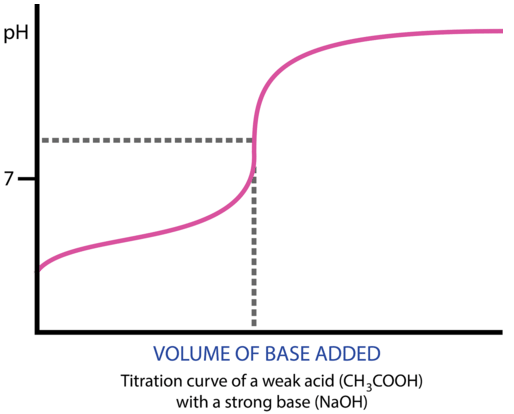# 7.20: Titration Curves

$$\newcommand{\vecs}{\overset { \rightharpoonup} {\mathbf{#1}} }$$ $$\newcommand{\vecd}{\overset{-\!-\!\rightharpoonup}{\vphantom{a}\smash {#1}}}$$$$\newcommand{\id}{\mathrm{id}}$$ $$\newcommand{\Span}{\mathrm{span}}$$ $$\newcommand{\kernel}{\mathrm{null}\,}$$ $$\newcommand{\range}{\mathrm{range}\,}$$ $$\newcommand{\RealPart}{\mathrm{Re}}$$ $$\newcommand{\ImaginaryPart}{\mathrm{Im}}$$ $$\newcommand{\Argument}{\mathrm{Arg}}$$ $$\newcommand{\norm}{\| #1 \|}$$ $$\newcommand{\inner}{\langle #1, #2 \rangle}$$ $$\newcommand{\Span}{\mathrm{span}}$$ $$\newcommand{\id}{\mathrm{id}}$$ $$\newcommand{\Span}{\mathrm{span}}$$ $$\newcommand{\kernel}{\mathrm{null}\,}$$ $$\newcommand{\range}{\mathrm{range}\,}$$ $$\newcommand{\RealPart}{\mathrm{Re}}$$ $$\newcommand{\ImaginaryPart}{\mathrm{Im}}$$ $$\newcommand{\Argument}{\mathrm{Arg}}$$ $$\newcommand{\norm}{\| #1 \|}$$ $$\newcommand{\inner}{\langle #1, #2 \rangle}$$ $$\newcommand{\Span}{\mathrm{span}}$$$$\newcommand{\AA}{\unicode[.8,0]{x212B}}$$

The $$x$$-$$y$$ plot that we know of as a graph was the brainchild of the French mathematician-philosopher Rene Descartes (1596-1650). His studies in mathematics led him to develop what was known as "Cartesian geometry", including the concept of today's graphs. The coordinates are often referred to as Cartesian coordinates.

## Titration Curves

As base is added to acid at the beginning of a titration, the pH rises very slowly. Nearer to the equivalence point, the pH begins to rapidly increase. If the titration is a strong acid with a strong base, the pH at the equivalence point is equal to 7. A bit past the equivalence point, the rate of change of the pH again slows down. A titration curve is a graphical representation of the pH of a solution during a titration. The figure below shows two different examples of a strong acid-strong base titration curve. On the left is a titration in which the base is added to the acid, and so the pH progresses from low to high. On the right is a titration in which the acid is added to the base. In this case, the pH starts out high and decreases during the titration. In both cases, the equivalence point is reached when the moles of acid and base are equal and the pH is 7. This also corresponds to the color change of the indicator.Figure $$\PageIndex{1}$$: A titration curve shows the pH changes that occur during the titration of an acid with a base. On the left, base is being added to acid. On the right, acid is being added to base. In both cases, the equivalence point is at pH 7. (CC BY-NC; CK-12)

Titration curves can also be generated in the case of a weak acid-strong base titration or a strong acid-weak base titration. The general shape of the titration curve is the same, but the pH at the equivalence point is different. In a weak acid-strong base titration, the pH is greater than 7 at the equivalence point. In a strong acid-weak base titration, the pH is less than 7 at the equivalence point.Figure $$\PageIndex{2}$$: Titration curve of weak acid and strong base. (CC BY-NC; CK-12)

## Summary

• A titration curve is a graphical representation of the pH of a solution during a titration.
• In a strong acid-strong base titration, the equivalence point is reached when the moles of acid and base are equal and the pH is 7.
• In a weak acid-strong base titration, the pH is greater than 7 at the equivalence point.
• In a strong acid-weak base titration, the pH is less than 7 at the equivalence point.

7.20: Titration Curves is shared under a CC BY-NC license and was authored, remixed, and/or curated by LibreTexts.